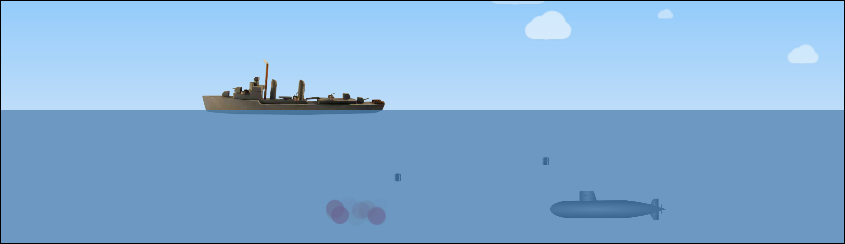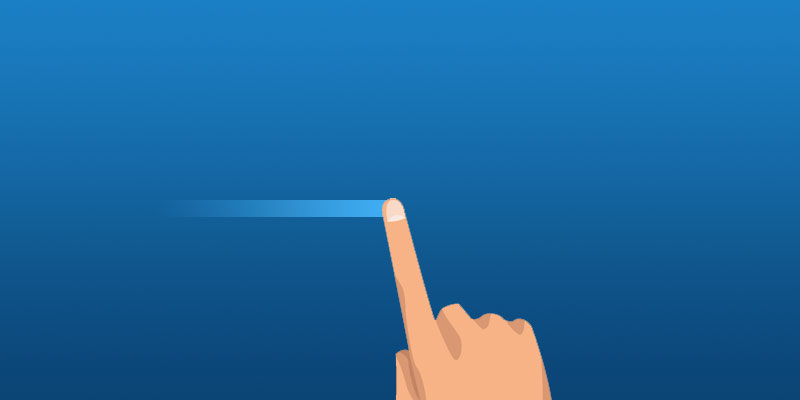↓ I built a game! Check it out ↓Capture Touch Events With JavaScript

June 19, 2018 by Andreas WikWhatever brilliant little project it is you’re working on, this method below should have you covered capturing swipes and taps.

Let’s first create a tiny bit of markup – the area in which you want to capture the events. In this case I’ll just give the body an ID and use that.

``<body id="the-body"></body>``

``````let pageWidth = window.innerWidth || document.body.clientWidth;
let threshold = Math.max(1, Math.floor(0.01 * (pageWidth)) );

let touchstartX = 0;
let touchstartY = 0;
let touchendX = 0;
let touchendY = 0;

const limit = Math.tan(45 * 1.5 / 180 * Math.PI);
const gestureZone = document.getElementById('the-body');``````

As you can see, first of all we get the width of the document.

Next, we create a threshold value which will determine if the user has actually swiped or just tapped. If the user has moved their finger tip less than the threshold, it’s a tap, otherwise it’s a swipe.

We also set a limit which will help us determine whether the user swipes diagonally or vertically.

Finally, grab the area on which the touch events will be captured – in this case the body.

Let’s set up a couple of event listeners, one for when the user starts touching (oh la la) and another for when they let go. Once letting go, we’ll run a function called handleGesture() which does the actual work.

``````gestureZone.addEventListener('touchstart', function(event) {
touchstartX = event.changedTouches.screenX;
touchstartY = event.changedTouches.screenY;
}, false);

touchendX = event.changedTouches.screenX;
touchendY = event.changedTouches.screenY;
handleGesture(event);
}, false);``````

Pretty straightforward. We add the event listeners to our gestureZone (the body, remember?) and grab X and Y coordinates of where on the screen the gesture started and where it ended.

Once the touch event/gesture has finished, we run this function:

``````function handleGesture(e) {

let x = touchendX - touchstartX;
let y = touchendY - touchstartY;
let xy = Math.abs(x / y);
let yx = Math.abs(y / x);

if (Math.abs(x) > threshold || Math.abs(y) > threshold) {

// Swipe

}
else {

// Tap
}
}``````

What’s happening here? First, we check if the pixels moved horizontally or vertically are larger than the threshold, meaning it was a swipe. Otherwise it was a tap.

Let’s finalize the function with the last pieces, deciding if it’s a left, right, up or down swipe. All needed for that is comparing the X/Y movement with the limit variable.

``````function handleGesture(e) {

let x = touchendX - touchstartX;
let y = touchendY - touchstartY;
let xy = Math.abs(x / y);
let yx = Math.abs(y / x);

if (Math.abs(x) > threshold || Math.abs(y) > threshold) {

if (yx <= limit) {

if (x < 0) {

console.log("swipe left");
}
else {

console.log("swipe right");
}
}
if (xy <= limit) {

if (y < 0) {
console.log("swipe up");
}
else {
console.log("swipe down");
}
}
}
else {
console.log("tap");
}
}``````

Working CodePen – try it out for yourself on your touch device:

See the Pen Capture Touch Screen Swipe and Tap Events by Andreas Wik (@andreaswik) on CodePen.